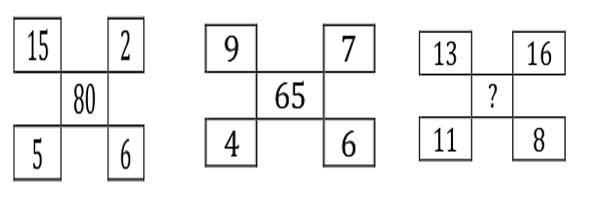Question 16

# Insert the missing numberSolution

Middle number = (Difference between the numbers written on left hand side)*(Sum of the numbers written on right hand side)

80 = (15 - 5)*(2 + 6)

65 = (9 - 4)*(7 + 6)

Therefore, ? = (13 - 11)*(16 + 8) = 2*24 = 48.

Hence, option B is the correct answer.